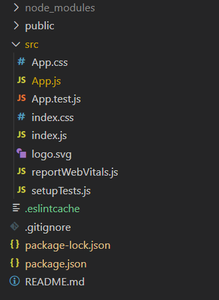# How to lift state two levels up in ReactJS ?

• Last Updated : 08 Apr, 2021

We want to lift the state two levels up such that when an event occurs at the lower level component then it should affect the state two levels up. For example, there are three components X, Y, Z. X is the parent of Component Y, Component Y is the parent of Component Z.

When some event occurs in component Z then it should affect the state of component X that is two-level up from component Z. for this we have to pass a function as a prop from component X to component Y then component Y will pass this function to component X so that Component X can call that function and change the state of component X.

Example:

• State of X

`this.state={ stateA: "whatever"}`
• Function in X:

```setStateX(newValue){
this.setState({stateA: newValue});
}```
• Now pass it as a prop to Y

`<Y setStateX={this.setStateX} />`
• In Y:

`<Z setStateX={this.props.setStateX} />`
• In Z:

`this.props.setStateX("new value of stateX");`

Call the function to set the state of X.

Creating React Application:

Step 1: Create a React application using the following command:

`npx create-react-app foldername`

Step 2: After creating your project folder i.e. foldername, move to it using the following command:

`cd foldername`

Project Structure: It will look like the following.Example:

## App.js

 `import React from ``'react'`` ` `class X extends React.Component {`` ` `    ``state = {``        ``name:``"Kapil"``    ``}`` ` `    ``setStateX = (newState) => {``        ``this``.setState({name:newState})``    ``}``     ` `    ``render() {``        ``return` `(``        ``
``        ``

Hi my name is { ``this``.state.name }

``        ````        ``
``        ``);``    ``}``     ` `}``class Y extends React.Component {`` ` `    ``render() {``        ``return``(``            ``
``                ````            ``
``        ``);``    ``}``}``class Z extends React.Component {``    ``render() {``        ``return``(``            ``
``                ````            ``
``        ``)``    ``}``}``export ``default` `X;`

Output:My Personal Notes arrow_drop_up